# Test: 35 Year JEE Previous Year Questions: The s-Block Elements

## 24 Questions MCQ Test Chemistry 35 Years JEE Main & Advanced Past year Papers | Test: 35 Year JEE Previous Year Questions: The s-Block Elements

Description
Attempt Test: 35 Year JEE Previous Year Questions: The s-Block Elements | 24 questions in 50 minutes | Mock test for JEE preparation | Free important questions MCQ to study Chemistry 35 Years JEE Main & Advanced Past year Papers for JEE Exam | Download free PDF with solutions
QUESTION: 1

### Read the following statement and explanation and answer as per the options given below : Statement : The alkali metals can form ionic hydrides which contain the hydride ion H–. Explanation : The alkali metals have low electronegativity; their hydrides conduct electricity when fused and liberate hydrogen at the anode.

Solution:

Both S & E are true and E is the correct explanation of S.

QUESTION: 2

### This question contains STATEMENT-1 (Assertion) and STATEMENT-2 (Reason) and has 4 choices (a), (b), (c) and (d) out of which ONLY ONE is correct. STATEMENT-1 :  Alkali metals dissolve in liquid ammonia to give blue solutions. because STATEMENT-2 : Alkali metals is liquid ammonia give solvated species of the type [M(NH3)n]+ (M = alkali metals).

Solution:

Statement-1 is correct. Statement-2 is also correct but not the correct explanation becuase blue colour of the solution is due to the solvated electrons.

QUESTION: 3

### KO2 (potassium super oxide) is used in oxygen cylinders in space and submarines because it

Solution:

2KO2 + 2H2O → 2 KOH + H2O2 + O2.
KO2 is used as an oxidising agent. It is used as air purifier in space capsules. Submarines and breathing masks as it produces oxygen and remove carbon dioxide.

QUESTION: 4

The metallic sodium disolves in liquid ammonia to form a deep blue coloured solution. The deep blue colour is due to formation of:

Solution:

The alkali metals dissolve in liquid ammonia without evolution of hydrogen. The metal loses electrons and combine with ammonia molecule.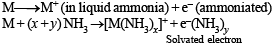It is ammoniated electron which is responsible for colour.

QUESTION: 5

A metal M readily forms its sulphate MSO4 which is watersoluble. It forms its oxide MO which becomes inert on heating. It forms an insoluble hyroxide M(OH)2 which is soluble in NaOH solution. Then M is

Solution:

Sulphate of alkaline earth metal are sparingly soluble or almost not soluble in water whereas BeSO4 is soluble in water due to high degree of solvation. Be(OH)2 is insoluble in water but soluble in NaOH.

BeO + 2NaOH→ Na2BeO2 + H2O

QUESTION: 6

In curing cement plasters water is sprinkled from time to time. This helps in

Solution:

Setting of cement is exothermic process which develops interlocking crystals of hydrated silicates

QUESTION: 7

The substance not likely to contain CaCO3 is

Solution:

Gypsum is CaSO4.2H2O

QUESTION: 8

The solubilities of carbonates decrease down the magnesium group due to a decrease in

Solution:

As we move down the group, the lattice energies of carbonates remain approximately the same. However the hydration energies of the metal cation decreases from Be++ to Ba++, hence the solubilities of carbonates of the alkaline earth metal decrease down the group mainly due to decreasing hydration energies of the cations  from Be++ to Ba++.

QUESTION: 9

Which one of the following processes will produce hard water ?

Solution:

Permanent hardness of water is due to chlorides and sulphates of calcium and magnesium i.e CaCl2, CaSO4, MgCl2 and MgSO4.

QUESTION: 10

One mole of magnesium nitride on the reaction with an excess of water gives :

Solution: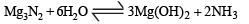QUESTION: 11

Which of the following species is diamagnetic in nature?

Solution:

TIPS/Formulae :
A diamagnetic substance contains no unpaired electron.
H2 is diamagnetic as it contains all paired electrons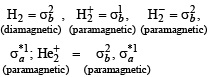QUESTION: 12

Based on lattice energy and other considerations which one of the following alkali metal chlorides is expected to have the highest melting point ?

Solution:

LiCl has partly covalent character. Other halides are ionic  in nature. Lattice energy decreases with increase of ionic radius of cation, anion being the same. Larger is the lattice energy, the higher will be m. pt. hence NaCl will have highest lattice energy.

QUESTION: 13

Which of the following statements in relation to the hydrogen atom is correct ?

Solution:

NOTE : In one electron species, such as H-atom, the energy of orbital depends only on the principal quantum number, n.

i.e. is < 2s = 2p < 3s = 3p = 3d < 4s = 4p ⇒ 4d = 4g

QUESTION: 14

The ionic mobility of alkali metal ions in aqueous solution is maximum for

Solution:

Smaller the size of cation higher is its hydration energy and greater is its ionic mobility hence the correct order is Li+ < Na+ < K+ <  Rb+

QUESTION: 15

In context with the industrial preparation of hydrogen from water gas (CO + H2), which of the following is the correct statement?

Solution:

On the industrial scale hydrogen is prepared from water gas according to following reaction sequence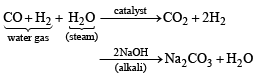From the above it is clear that CO is first oxidised to CO2 which is then absorbed in NaOH.

QUESTION: 16

Which of the following on thermal decomposition yields a basic as well as acidic oxide ?

Solution:

Calcium carbonate on thermal decomposition gives CaO (Basic oxide) and CO2 (Acidic oxide)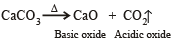QUESTION: 17

Very pure hydrogen (99.9) can be made by which of the following processes ?

Solution:

Very pure hydrogen can be prepared by the action of water on sodium hydride.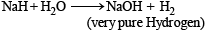QUESTION: 18

In which of the following reactions H2O2 acts as a reducing agent?

Solution:

The reducing agent loses electron during redox reaction i.e. oxidises itself.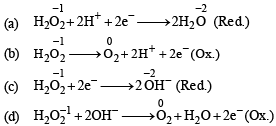QUESTION: 19

Which one of the following alkaline earth metal sulphates has its hydration enthalpy greater than its lattice enthalpy ?

Solution:

In alkaline earth metals, ionic size increases down the group. The lattice energy remains constant because sulphate  ion is so large, so that small change in cationic size does not make any difference. On moving down the group the degree of hydration of metal ions decreases very much leading to decrease in solubility.

∴ BeSO4 > MgSO4 > CaSO4 > SrSO4 > BaSO4

QUESTION: 20

The molecular formula of a commercial resin used for exchanging ions in water softening is C8H7SO3 Na+ (Mol. wt. 206. What would be the maximum uptake of Ca2+ ions by the resin when expressed in mole per gram resin ?

Solution:

2 mole of water softner require 1 mole of Ca2+ ion

So, 1 mole of water softner require 1/2 mole of Ca2+ ion

Thus,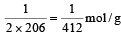will be maximum uptake

QUESTION: 21

From the following statements regarding H2O2, choose the incorrect statement :

Solution:

H2O2 has oxidizing and reducing properties both.

QUESTION: 22

Which one of the following statements about water is FALSE?

Solution:

There  is  extensive  intermolecular hydrogen bonding in  the  condensed phase  instead  of intramolecular H-bonding.

QUESTION: 23

Which of the following atoms has the highest first ionization energy?

Solution:

Alkali metals have the lowest ionization energy in each period on the other hand Sc is a d - block element.
Transition metals have smaller atomic radii and higher nuclear charge leading to high ionisation energy.

QUESTION: 24

The main oxides formed on combustion of Li, Na and K in excess of air are, respectively:

Solution:

On heating with excess of air Li, Na and K forms following oxides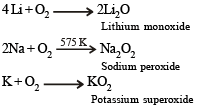Use Code STAYHOME200 and get INR 200 additional OFF Use Coupon Code